Homework Help Question & Answers

# For nickel, Ni, the heat of vaporization at its normal boiling point of 2732 OC is...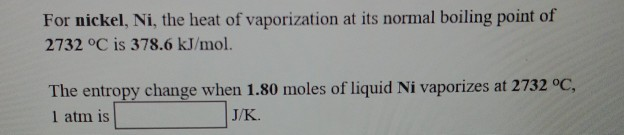For nickel, Ni, the heat of vaporization at its normal boiling point of 2732 OC is 378.6 kJ/mol. The entropy change when 1.80 moles of liquid Ni vaporizes at 2732 oc, 1 atm is J/K.

#### Homework Answers

Answer #1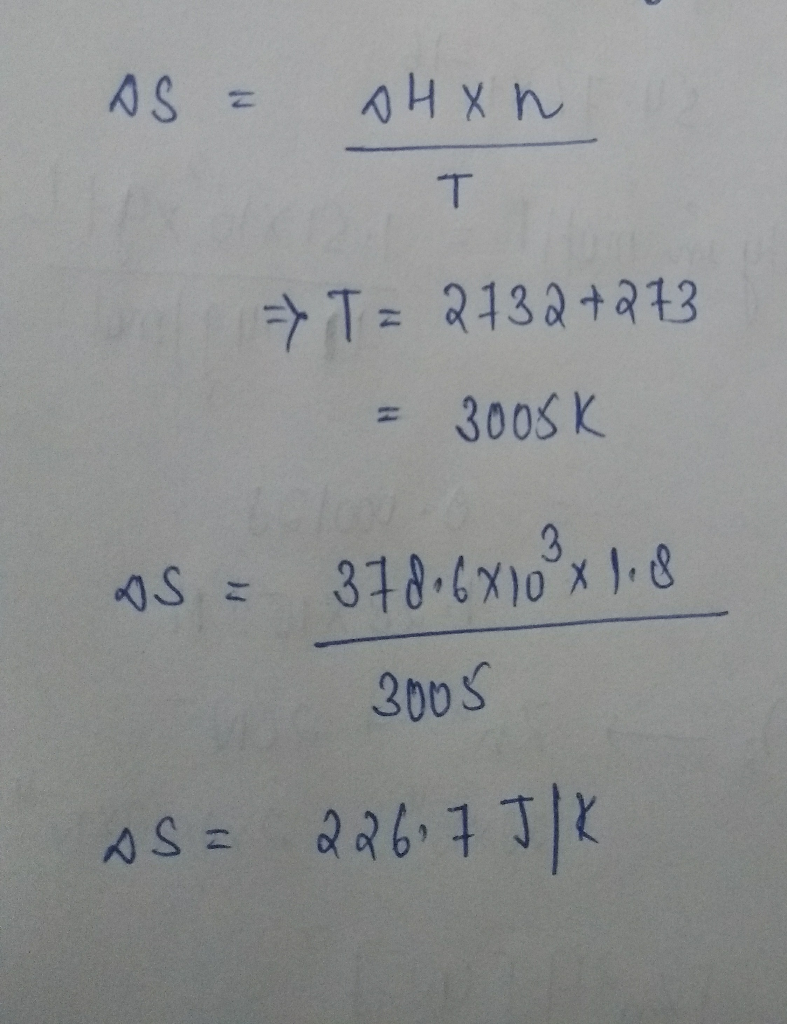Know the answer?
Your Answer:

#### Post as a guest

Your Name:

What's your source?

#### Earn Coin

Coins can be redeemed for fabulous gifts.

Not the answer you're looking for? Ask your own homework help question. Our experts will answer your question WITHIN MINUTES for Free.
Similar Homework Help Questions
• ### For cobalt, Co, the heat of vaporization at its normal boiling point of 3097 °C is...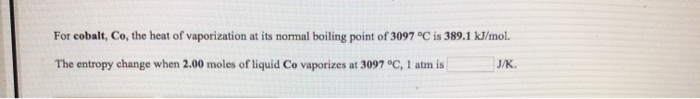For cobalt, Co, the heat of vaporization at its normal boiling point of 3097 °C is 389.1 kJ/mol. The entropy change when 2.00 moles of liquid Co vaporizes at 3097 °C, 1 atm is J/K

• ### For bismuth, Bi, the heat of vaporization at its normal boiling point of 1627 °C is...

For bismuth, Bi, the heat of vaporization at its normal boiling point of 1627 °C is 172.0 kJ/mol. The entropy change when 2.11 moles of Bi vapor condenses at 1627 °C, 1 atm is J/K.

• ### For silicon, Si, the heat of vaporization at its normal boiling point of 2355 °C is...

For silicon, Si, the heat of vaporization at its normal boiling point of 2355 °C is 297.1 kJ/mol. The entropy change when 2.26 moles of Si vapor condenses at 2355 °C, 1 atm is J/K.

• ### the normal boiling point of ethanol is 78.3 deg C and its molar enthalpy of vaporization is 38.56 Kj/mol. what is the ch...

the normal boiling point of ethanol is 78.3 deg C and its molar enthalpy of vaporization is 38.56 Kj/mol. what is the change in entropy in the system in J/k when 97.2 grams of ethanol at 1 atm condenses to a liquid at the normal boiling point?

• ### PLEASE ANSWER ALL 3 QUESTIONS on entropy change and SHOW WORK?! Use the References to access...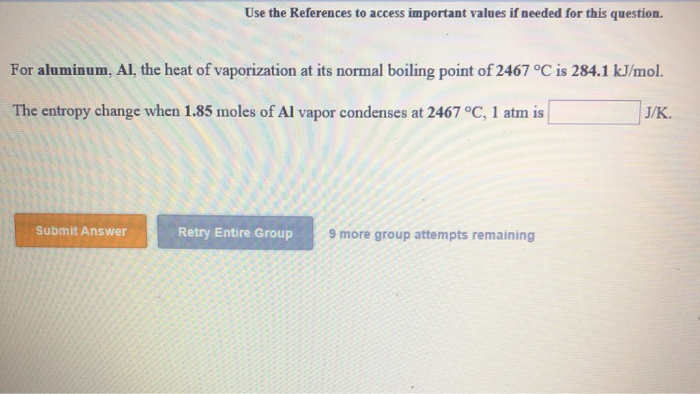PLEASE ANSWER ALL 3 QUESTIONS on entropy change and SHOW WORK?! Use the References to access important values if needed for this question. For aluminum, Al, the heat of vaporization at its normal boiling point of 2467 °C is 284.1 kJ/mol. The entropy change when 1.85 moles of Al vapor condenses at 2467 °C, 1 atm is J/K. Submit Answer Retry Entire Group 9 more group attempts remaining Use the References to access important values if needed for this question....

• ### 14. For bismuth, Bi, the heat of fusion at its normal melting point of 271 °C...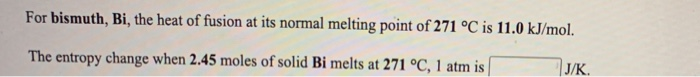14. For bismuth, Bi, the heat of fusion at its normal melting point of 271 °C is 11.0 kJ/mol. The entropy change when 2.45 moles of solid Bi melts at 271 °C, 1 atm is J/K. For magnesium, Mg, the heat of fusion at its normal melting point of 649 °C is 9.0 kJ/mol. The entropy change when 1.72 moles of liquid Mg freezes at 649 °C, 1 atm is J/K

• ### The normal boiling point of liquid octane is 399 K. Assuming that its molar heat of...

The normal boiling point of liquid octane is 399 K. Assuming that its molar heat of vaporization is constant at 36.3 kJ/mol, the boiling point of C8H18 when the external pressure is 1.30 atm is __________K.

• ### The normal boiling point of liquid butanol is 391 K. Assuming that its molar heat of...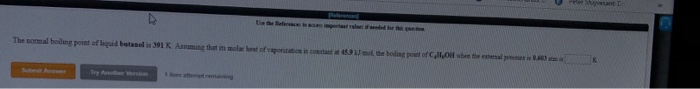The normal boiling point of liquid butanol is 391 K. Assuming that its molar heat of vaporization is constant at 45.9 kJ/mol, the boiling point of C4H9OHwhen the external pressure is 0.603 atm is ________ K.

• ### Use the References to access important values if needed for this question. The normal boiling point...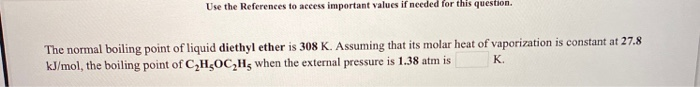Use the References to access important values if needed for this question. The normal boiling point of liquid diethyl ether is 308 K. Assuming that its molar heat of vaporization is constant at 27.8 kJ/mol, the boiling point of C,H,OC Hz when the external pressure is 1.38 atm is K.

• ### The enthalpy of vaporization of trichloromethane (chloroform, CHC13) is 29.4 kJ mol-'at its normal boiling point...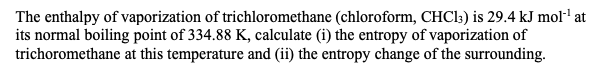The enthalpy of vaporization of trichloromethane (chloroform, CHC13) is 29.4 kJ mol-'at its normal boiling point of 334.88 K, calculate (i) the entropy of vaporization of trichoromethane at this temperature and (ii) the entropy change of the surrounding.